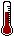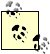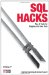# Hack 82. Generate Sequential or Missing DataYou can use an integers table to generate sequential data, or provide missing data in an OUTER JOIN.

What is an integers table? Sometimes called a numbers or sequence table, an integers table is simply a table containing some integers. It's not a system table; it's a user table.A user table is one that you have to create, because it doesn't come preinstalled with the database system. If you work in a team environment, ask around to see whether someone else has an integers table, and find out how they use it.

An integers table allows you to write queries which involve a range of values. Queries can use an integers table to do things such as:

• Generate sequential data
• Provide missing data in an OUTER JOIN

10.6.1. Create and Populate an integers Table

Your integers table should have a single column called i, which is defined as the table's primary key:

```CREATE TABLE integers
( i INTEGER NOT NULL PRIMARY KEY )```

Declaring i as the primary key guarantees you cannot accidentally populate the table twice. It also defines an index, which helps for optimization.

You can start by inserting the digits 0 through 9:

```INSERT
INTO integers (i)
VALUES
(0),(1),(2),(3),(4),(5),(6),(7),(8),(9);```

```INSERT INTO integers (i) VALUES (0);
INSERT INTO integers (i) VALUES (1);
INSERT INTO integers (i) VALUES (2);
INSERT INTO integers (i) VALUES (3);
INSERT INTO integers (i) VALUES (4);
INSERT INTO integers (i) VALUES (5);
INSERT INTO integers (i) VALUES (6);
INSERT INTO integers (i) VALUES (7);
INSERT INTO integers (i) VALUES (8);
INSERT INTO integers (i) VALUES (9);```

10.6.2. Generate Sequential Data

You may be wondering how useful the integers 0 through 9 can be. Watch carefully; this may seem like magic, but it isn't.

10.6.2.1. Numbers 099

Because the integers table has only the numbers 0 through 9, if you want more numbers, you need to do something more. So simply CROSS JOIN the integers table to itself, as in this query:

```SELECT 10*t.i+u.i AS number
FROM integers AS u
CROSS
JOIN integers AS t
ORDER
BY number
;```

In a CROSS JOIN, every row of one table is joined to every row of the other. If your database system doesn't support CROSS JOIN syntax, use this instead:

```SELECT 10*t.i+u.i AS number
FROM integers AS u
, integers AS t
ORDER
BY number;```You may recognize the preceding code as an "old-style" join which is missing its WHERE clause. That's how we did cross-joins before the CROSS JOIN syntax was invented. If you've ever accidentally omitted the WHERE clause in this type of join, you already know what cross-join effects are.

Because the integers table is used as both tables, this is a self-join, which requires using table aliases. The alias names t and u stand for tens and units. The expression 10 * t.i + u.i involves a calculation using the value of i from each table, and we give this expression the column alias name number. Finally, the result set is sorted by number:

```number
+------+
| 0 |
| 1 |
| 2 |
... |
| 99 |
+------+

100 rows in set```

10.6.2.2. Letters AZ

You can also use the integers table to generate the alphabet:

```SELECT SUBSTRING('ABCDEFGHIJKLMNOPQRSTUVWXYZ'
FROM 10*t.i+u.i FOR 1) AS letter
FROM integers u
CROSS
JOIN integers t
WHERE 10*t.i+u.i BETWEEN 1 AND 26
ORDER
BY letter;```

The query uses the expression 10 * t.i + u.i as the value of one of the parameters in the SUBSTRING function. Instead of 0 through 99, as in the preceding query, the integers are restricted to 1 through 26 by a condition in the WHERE clause:

```letter
A
B
C
...
Z
26 rows in set```

10.6.2.3. SQL Server

In SQL Server, you separate the parameters of the SUBSTRING function with commas:

```SELECT SUBSTRING('ABCDEFGHIJKLMNOPQRSTUVWXYZ'
, 10*t.i+u.i , 1) AS letter
FROM integers u
CROSS
JOIN integers t
WHERE 10*t.i+u.i BETWEEN 1 AND 26
ORDER
BY letter```

10.6.2.4. Oracle

You need to use SUBSTR in Oracle:

```SELECT SUBSTR('ABCDEFGHIJKLMNOPQRSTUVWXYZ'
, 10*t.i+u.i , 1) AS letter
FROM integers u
CROSS
JOIN integers t
WHERE 10*t.i+u.i BETWEEN 1 AND 26
ORDER
BY letter```

10.6.2.5. Date ranges

You can even use the integers table to generate a sequence of dates:

```SELECT current_date
+ INTERVAL 10*t.i+u.i day AS next_30_dates
FROM integers u
CROSS
JOIN integers t
WHERE 10*t.i+u.i BETWEEN 0 AND 29
ORDER
BY next_30_dates;```

This query generates dates for the next 30 days, including today:

```next_30_dates
2008-02-27
2008-02-28
2008-02-29
...
2008-03-27
30 rows in set```

10.6.2.6. Oracle and PostgreSQL

You can use the same technique with Oracle and PostgreSQL:

```SELECT CURRENT_DATE
+ 10*t.i+u.i AS next_30_dates
FROM integers u
CROSS
JOIN integers t
WHERE 10*t.i+u.i BETWEEN 0 AND 29
ORDER
BY next_30_dates```

10.6.2.7. SQL Server

SQL Server needs the GEtdATE( ) function:

```SELECT GETDATE( )
+ 10*t.i+u.i AS next_30_dates
FROM integers u
CROSS
JOIN integers t
WHERE 10*t.i+u.i BETWEEN 0 AND 29
ORDER
BY next_30_dates```

10.6.3. Provide Missing Data in an OUTER JOIN

Now you can take your generated data and put it to work as the left table in a LEFT OUTER JOIN. The benefit of doing this is that data points which are missing from the data table (the right table) will be included in the result set.

10.6.3.1. Counts for each letter

Suppose you have a table of articles. Using a LEFT OUTER JOIN with your INTEGERS table to generate the letters A through Z, you can count the number of article titles for each letter, including a count for letters which have no corresponding article titles:

```SELECT letters.letter
, COUNT(articles.title) AS titles
FROM (
SELECT SUBSTRING('ABCDEFGHIJKLMNOPQRSTUVWXYZ'
FROM 10*t.i+u.i FOR 1) AS letter
FROM integers AS u
CROSS
JOIN integers AS t
WHERE 10*t.i+u.i BETWEEN 1 AND 26
) AS letters
LEFT OUTER
JOIN articles
ON letters.letter
= SUBSTRING(articles.title FROM 1 FOR 1)
GROUP
BY letters.letter```

Because it's a LEFT OUTER JOIN, all 26 letters will be included in the result set with a count of 0 if no article titles start with a particular letter:

```letter titles
A 1
B 9
C 37
...
Z 0
26 rows in set```

10.6.3.2. Data for consecutive dates

When generating summary data for graphing purposes, it's important that every date in the sample range be included, even if there are no sample values for a given date. This query uses a table, SAMPLES, which contains S_DATE (the date when the sample was taken) and S_VALUE (the value of the sample):

```SELECT dates.X_axis_date
, SUM(samples.s_value) AS Y_axis_value
FROM (
SELECT ( SELECT MIN(s_date) FROM samples )
+ INTERVAL 10*t.i+u.i day AS X_axis_date
FROM integers AS u
CROSS
JOIN integers AS t
WHERE ( SELECT min(s_date) FROM samples )
+ INTERVAL 10*t.i+u.i day
<= ( SELECT MAX(s_date) FROM samples )
) AS dates
LEFT OUTER
JOIN samples
ON dates.X_axis_date
= samples.s_date
GROUP
BY dates.X_axis_date```

Notice how instead of specifying fixed numbers in the WHERE clause, you let the range of dates between MIN and MAX in the sample data determine which dates to generate with the integers table. It might produce:

```X_axis_date Y_axis_value
2006-07-05 10
2006-07-06 10
2006-07-07 20
2006-07-08 NULL
2006-07-09 NULL
2006-07-10 50
6 rows```The assumption in the preceding query is that the range of dates is no greater than 100 days, because that's the most numbers that the expression 10 * t.i + u.i can produce. For more numbers, read on.

10.6.4. Hacking the Hack

You can simplify queries which use self-joins on the integers table by declaring views that incorporate the self-join. Here's a view for the first 100 integers:

```CREATE
VIEW hundred (i)
AS
SELECT 10*t.i+u.i
FROM integers u
CROSS
JOIN integers t```

Here's a view for the first 1,000 integers:

```CREATE
VIEW thousand (i)
AS
SELECT 100*h.i+10*t.i+u.i
FROM integers u
CROSS
JOIN integers t
CROSS
JOIN integers h```

Now if you need a range of dates to cover a period of days up to a year, you can use the thousand view:

```SELECT current_date
+ INTERVAL i day AS next_year_dates
FROM thousand
WHERE current_date
+ INTERVAL i day
< current_date
+ INTERVAL 1 year
ORDER
BY next_year_dates```

Instead of specifying a fixed number in the WHERE clause, this query allows the data to determine the range of dates. The result set will be either 365 dates or 366, depending on whether the date range includes a leap day:

```next_year_dates
2007-01-01
2007-01-02
2007-01-03
...
2007-12-31
365 rows

next_year_dates
2007-03-01
2007-03-02
2007-03-03
...
2008-02-29
366 rows```

10.6.4.1. Oracle

In Oracle, you can use the INTERVAL notation with a quoted number:

```SELECT current_date
+ i AS next_year_dates
FROM thousand
WHERE current_date
+ i
< current_date
+ INTERVAL '1' year
ORDER
BY next_year_dates```

10.6.4.2. PostgreSQL

In PostgreSQL, you quote the number and the word year:

```SELECT current_date
+ i AS next_year_dates
FROM thousand
WHERE current_date
+ i
< current_date
+ INTERVAL '1 year'
ORDER
BY next_year_dates```

10.6.4.3. SQL Server

In SQL Server, you can use the DateAdd function:

```SELECT GetDate( )
+ i AS next_year_dates
FROM thousand
WHERE GetDate( )
+ i
ORDER
BY next_year_dates```

Rudy LimebackSQL Hacks
ISBN: 0596527993
EAN: 2147483647
Year: 2004
Pages: 147